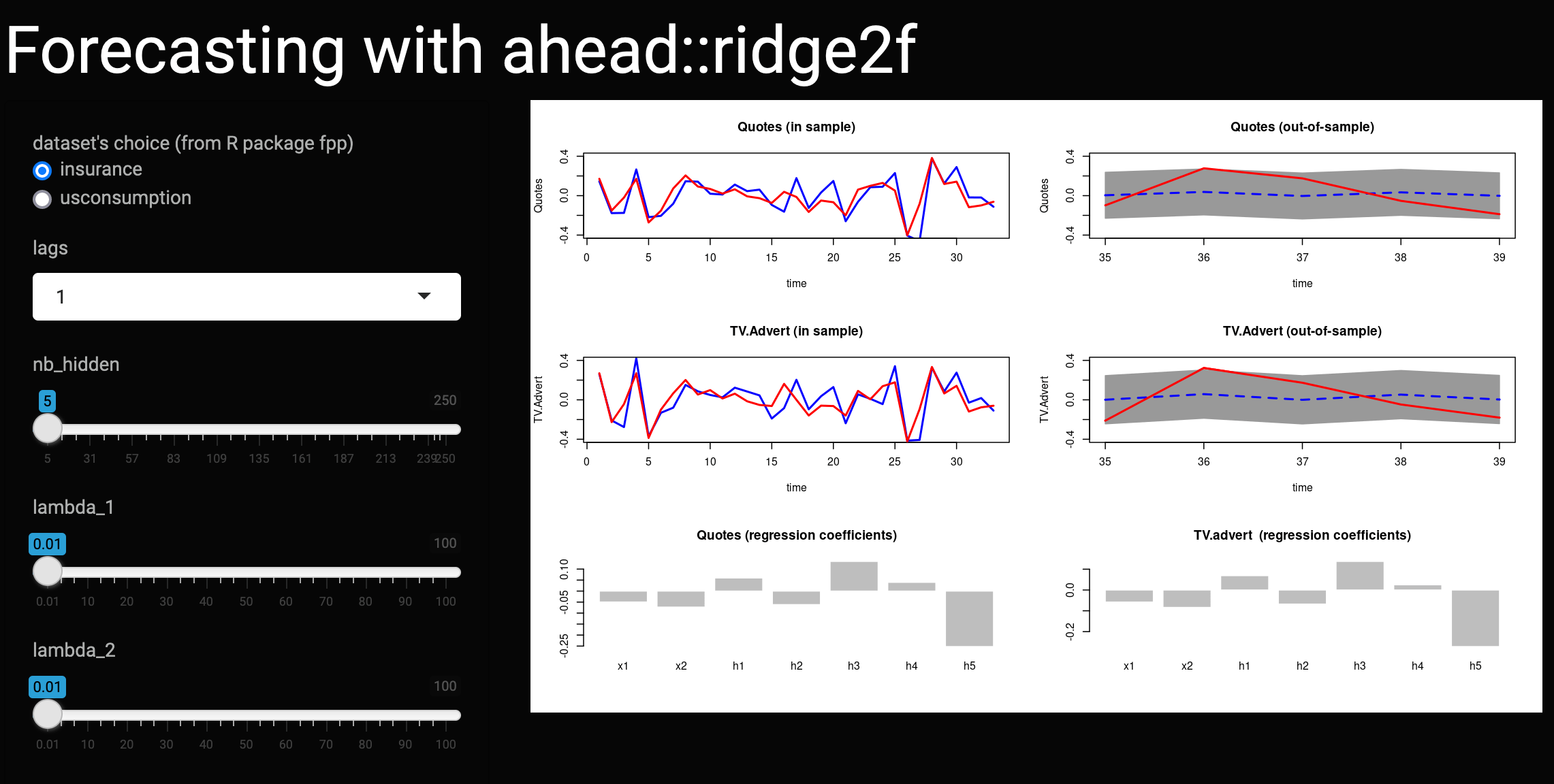This article was first published on T. Moudiki's Webpage - Python , and kindly contributed to python-bloggers. (You can report issue about the content on this page here)

Here is a link to a dashboard illustrating bivariate time series forecasting with the package ahead:

https://thierry.shinyapps.io/ridge2shiny/

This dashboard is more specifically about ahead::ridge2f (in R) and ahead.Ridge2Regressor (in Python) hyperparameters’ meaning and impact. In the first two rows of the figure, everything related to ahead::ridge2f and ahead.Ridge2Regressor is colored in blue, in-sample and out-of-sample, whereas input series’ observed values are colored in red. Here are a few things you could try:

• Illustrating overfitting: Leave every other parameter constant – to their default value. Set the number of lags to 3, and increase the number of nodes in the hidden layer nb_hidden. Observe what happens on the right (two first rows of the figure), when the input is perfectly fitted.
• Illustrating shrinkage: Leave every other parameter constant – to their default value. Increase $$\lambda_1$$, and observe the regression coefficients (third row of the figure) associated to the original features $$x_1, x_2, \ldots$$ being shrinked towards zero.
• Illustrating shrinkage 2: Leave every other parameter constant – to their default value. Increase $$\lambda_2$$, and observe the regression coefficients (third row of the figure) associated to the hidden layer $$h_1, h_2, \ldots$$ being shrinked towards zero.